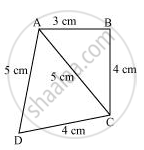Share

# Find the area of a quadrilateral ABCD in which AB = 3 cm, BC = 4 cm, CD = 4 cm, DA = 5 cm and AC = 5 cm. - Mathematics

Course

#### Question

Find the area of a quadrilateral ABCD in which AB = 3 cm, BC = 4 cm, CD = 4 cm, DA = 5 cm and AC = 5 cm.

#### SolutionFor ΔABC,

AC2 = AB2 + BC2

(5)2 = (3)2 + (4)2

Therefore, ΔABC is a right-angled triangle, right-angled at point B.

Area of ΔABC= 1/2xxABxxBC=1/2xx3xx4=6 cm^2

Perimeter = 2s = AC + CD + DA = (5 + 4 + 5) cm = 14 cm

s = 14/2 = 7 cm

By Heron’s formula,

"Area of triangle "=sqrt(s(s-a)(s-b)(s-c))

"Area of "triangle ADC=[sqrt(7(7-5)(7-5)(7-4))]cm^2

=(sqrt(7xx2xx2xx3))cm^2

=2sqrt21 cm^2

= (2 x 4.583) cm2

= 9.166 cm2

Area of ABCD = Area of ΔABC + Area of ΔACD

= (6 + 9.166) cm2

= 15.166 cm2

= 15.2 cm2 (approximately)

Is there an error in this question or solution?